# Class 11 Maths NCERT Solutions for Chapter 6 Linear Inequalities Exercise 6.1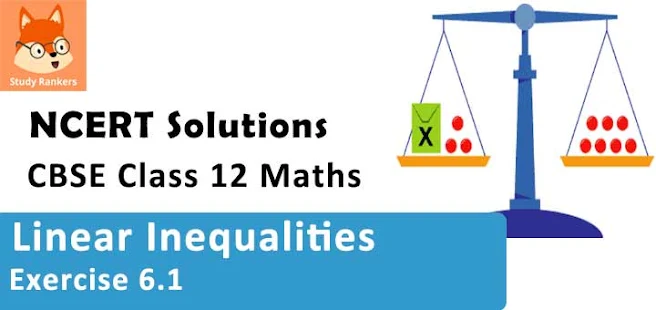### Linear Inequalities Exercise 6.1 Solutions

1. Solve 24x < 100, when

(i) x is a natural number

(ii) x is an integer.

Solution

The given inequality is 24x < 100.
24x < 100
⇒ 24x/24 < 100/24  [Dividing both sides by same positive number]
⇒ x < 25/6
(i) It is evident that 1, 2, 3, and 4 are the only natural numbers less than 25/6.
Thus, when x is a natural number, the solutions of the given inequality are 1, 2, 3, and 4.
Hence, in this case, the solution set is {1, 2, 3, 4}.
(ii) The integers less than 25/6 are …–3, –2, –1, 0, 1, 2, 3, 4.
Thus, when x is an integer, the solutions of the given inequality are …–3, –2, –1, 0, 1, 2, 3, 4.
Hence, in this case, the solution set is {…–3, –2, –1, 0, 1, 2, 3, 4}.

2. Solve –12x > 30, when
(i) x is a natural number
(ii) x is an integer

Solution

The given inequality is -12x > 30.
-12x > 30
⇒ -12x/-12 < 30/-12  [Dividing both sides by same negative number]
⇒ x < -5/2
(i) There is no natural number less than (-5/2).
Thus, when x is a natural number, there is no solution of the given inequality.
(ii) The integers less than (-5/2) are …, –5, –4, –3.
Thus, when x is an integer, the solutions of the given inequality are…, –5, –4, –3.
Hence, in this case, the solution set is {…, –5, –4, –3}.

3. Solve 5x - 3 < 7, when
(i) x is an integer
(ii) x is a real number

Solution

The given inequality is 5x - 3 < 7.
5x - 3 < 7
⇒ 5x - 3 + 3 < 7 + 3
⇒ 5x < 10
⇒ 5x/5 < 10/5
⇒ x < 2
(i) The integers less than 2 are…, –4, –3, –2, –1, 0, 1.
Thus, when x is an integer, the solutions of the given inequality are…, –4, –3, –2, –1, 0, 1.
Hence, in this case, the solution set is {…, –4, –3, –2, –1, 0, 1}.
(ii) When x is a real number, the solutions of the given inequality are given by x < 2, that is, all real numbers x which are less than 2.
Thus, the solution set of the given inequality is x ∈ (–∞, 2).

4. Solve 3x + 8 > 2, when
(i) x is an integer
(ii) x is a real number

Solution

The given inequality is 3x + 8 > 2.
3x + 8 > 2
⇒ 3x + 8 - 8 > 2 - 8
⇒ 3x > -6
⇒ 3x/3 > -6/3
⇒ x > -2
(i) The integers greater than –2 are –1, 0, 1, 2, …
Thus, when x is an integer, the solutions of the given inequality are –1, 0, 1, 2 …
Hence, in this case, the solution set is {–1, 0, 1, 2, …}.
(ii) When x is a real number, the solutions of the given inequality are all the real numbers, which are greater than –2.
Thus, in this case, the solution set is (– 2, ∞).

5. Solve the given inequality for real x : 4x + 3 < 5x + 7

Solution

4x + 3 < 5x + 7
⇒ 4x + 3 – 7 < 5x + 7 – 7
⇒ 4x – 4 < 5x
⇒ 4x – 4 – 4x < 5– 4x
⇒ –4 < x
Thus, all real numbers x,which are greater than –4, are the solutions of the given inequality.
Hence, the solution set of the given inequality is (–4, ∞).

6. Solve the given inequality for real x: 3x – 7 > 5x – 1

Solution

3x – 7 > 5x – 1
⇒ 3x – 7 + 7 > 5x – 1 + 7
⇒ 3x > 5x + 6
⇒ 3x – 5x > 5x + 6 – 5x
⇒ – 2> 6
⇒ -2x/-2 < 6/-2
⇒ x < -3
Thus, all real numbers x, which are less than -3, are the solutions of the given inequality.
Hence, the solution set of the given inequality is (-∞ , -3).

7. Solve the given inequality for real x: 3(x – 1) ≤ 2 (– 3)

Solution

3(x – 1) ≤ 2(x – 3)
⇒ 3x – 3 ≤ 2x – 6
⇒ 3x – 3 + 3 ≤ 2x – 6 + 3
⇒ 3x ≤ 2x – 3
⇒ 3x – 2≤ 2x – 3 – 2x
⇒ x ≤ – 3
Thus, all real numbers x,which are less than or equal to –3, are the solutions of the given inequality.
Hence, the solution set of the given inequality is (–∞, –3].

8. Solve the given inequality for real x: 3(2 – x) ≥ 2(1 – x)

Solution

3(2 – x) ≥ 2(1 – x)
⇒ 6 – 3x ≥ 2 – 2x
⇒ 6 – 3x + 2x ≥ 2 – 2+ 2x
⇒ 6 – x ≥ 2
⇒ 6 – x – 6 ≥ 2 – 6
⇒ –x ≥ –4
⇒ x ≤ 4
Thus, all real numbers x,which are less than or equal to 4, are the solutions of the given inequality.
Hence, the solution set of the given inequality is (–∞, 4].

9. Solve the given inequality for real x : x + x/2 + x/3 < 11

Solution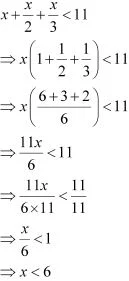Thus, all real numbers x,which are less than 6, are the solutions of the given inequality.
Hence, the solution set of the given inequality is (–∞, 6).

10. Solve the given inequality for real x : x/3 > x/2 +  1
Solution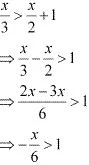⇒ -x >  6
⇒ x < -6
Thus, all real numbers x, which are less than –6, are the solutions of the given inequality.
Hence, the solution set of the given inequality is (–∞, –6).

11. Solve the given inequality for real x : 3(x-2)/5 ≤ 5(2-x)/3
Solution
3(x-2)/5 ≤ 5(2-x)/3
⇒ 9(x - 2) ≤  25(2-x)
⇒ 9x - 18 ≤ 50 - 25x
⇒ 9x - 18 + 25x ≤ 50
⇒ 34x - 18  ≤ 50
⇒ 34x ≤ 50 + 18
⇒ 34x ≤ 68
⇒ 34x/34 ≤ 68/34
⇒ x ≤ 4
Thus, all real numbers x,which are less than or equal to 2, are the solutions of the given inequality.
Hence, the solution set of the given inequality is (–∞, 2].

12. Solve the given inequality for real x : (1/2)(3x/5 + 4) ≥ (1/3)(x - 6)
Solution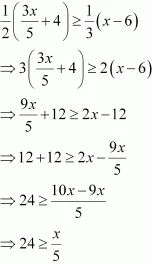⇒ 120 ≥ x
Thus, all real numbers x, which are less than or equal to 120, are the solutions of the given inequality.
Hence, the solution set of the given inequality is (-∞, 120).

13. Solve the given inequality for real x: 2(2x + 3) – 10 < 6 (x – 2)
Solution
2(2x+ 3) - 10< 6(x - 2)
⇒ 4x + 6 - 10 < 6x - 12
⇒ 4x -4 < 6x - 12
⇒ -4 + 12 < 6x - 4x
⇒ 8 < 2x
⇒ 4 < x
Thus, all real numbers x, which are greater than or equal to 4, are the solutions of the given inequality.
Hence, the solution set of the given inequality is (4, ∞).

14. Solve the given inequality for real x: 37 ­– (3x + 5) ≥ 9x – 8(– 3)
Solution
37 - (3x + 5) ≥ 9x - 8(x - 3)
⇒ 37 - 3x - 5 ≥ 9x - 8x + 24
⇒ 32 - 3x ≥ x + 24
⇒ 32  - 24 ≥ x + 3x
⇒ 8 ≥ 4x
⇒ 2 ≥ x
Thus, all real numbers x, which are less than or equal to 2, are the solutions of the given inequality.
Hence, the solution set of the given inequality is (–∞, 2].

15. Solve the given inequality for real x : x/4 <(5x - 2)/3 - (7x - 3)/5
Solution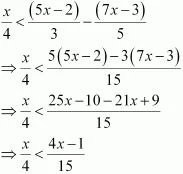⇒ 15x < 4(4x - 1)
⇒ 15x < 16x - 4
⇒ 4<16x - 15x
⇒ 4 < x
Thus, all real numbers x, which are greater than 4, are the solutions of the given inequality.
Hence, the solution set of the given inequality is (4, ∞).

16.  Solve the given inequality for real x : (2x -1)/3 ≥ (3x - 2)/4  - (2-x)/5
Solution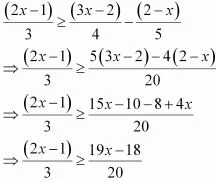⇒ 20(2x - 1) ≥ 3(19x - 18)
⇒ 40x - 20 ≥ 57x - 54
⇒ -20 + 54 ≥ 57x - 40x
⇒ 34 ≥ 17x
⇒ 2 ≥ x
Thus, all real numbers x,which are less than or equal to 2, are the solutions of the given inequality.
Hence, the solution set of the given inequality is (–∞, 2].

17. Solve the given inequality and show the graph of the solution on number line: 5x – 3 ≥ 3x – 5
Solution
5x - 3 ≥ 3x - 5
⇒ 5x - 3x ≥ -5 + 3
⇒ 2x ≥ - 2
⇒ 2x/2 ≥ -2/2
⇒ x ≥ -1
The graphical representation of the solutions of the given inequality is as follows .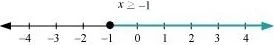18. Solve the given inequality and show the graph of the solution on number line: 3x – 2 < 2x +1
Solution

3x – 2 < 2x +1
⇒ 3– 2x < 1 + 2
⇒ x < 3
The graphical representation of the solutions of the given inequality is as follows.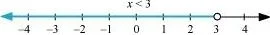19. Solve the given inequality and show the graph of the solution on number line: 3(1 – x) < 2 (x + 4).

Solution

3(1 – x) < 2 (x + 4)
⇒ 3 - 3x < 2x + 8
⇒ 3 - 8 < 2x + 3x
⇒ -5 < 5x
⇒ -5/5 < 5x/5
⇒ -1 < x
The graphical representation of the solutions of the given inequality is as follows .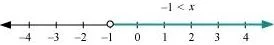20. Solve the given inequality and show the graph of the solution on number line : x/2 ≥ (5x - 2)/3 - (7x -3)/5

Solution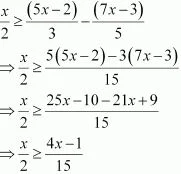⇒ 15x  ≥ 2(4x - 1)
⇒ 15x  ≥ 8x - 2
⇒ 15x - 8x  ≥ 8x - 2 - 8x
⇒ 7x  ≥ -2
⇒ x ≥ -2/7
The graphical representation of the solutions of the given inequality is as follows :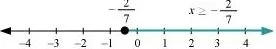21. Ravi obtained 70 and 75 marks in first two unit test. Find the minimum marks he should get in the third test to have an average of at least 60 marks.
Solution
Let x be the marks obtained by Ravi in the third unit test.
Since the student should have an average of at least 60 marks,
(70 + 75 + x)/3 ≥ 60
⇒ 145 + x ≥ 180
⇒ x ≥ 180 - 145
⇒ x ≥ 35
Thus, the student must obtain a minimum of 35 marks to have an average of at least 60 marks.

22. To receive Grade ‘A’ in a course, one must obtain an average of 90 marks or more in five examinations (each of 100 marks). If Sunita’s marks in first four examinations are 87, 92, 94 and 95, find minimum marks that Sunita must obtain in fifth examination to get grade ‘A’ in the course.
Solution

Let x be the marks obtained by Sunita in the fifth examination.
In order to receive grade ‘A’ in the course, she must obtain an average of 90 marks or more in five examinations.
Therefore,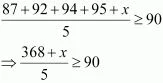⇒ 368 + x  ≥ 450
⇒ x  ≥ 450 - 368
⇒ x  ≥ 82
Thus, Sunita must obtain greater than or equal to 82 marks in the fifth examination.

23. Find all pairs of consecutive odd positive integers both of which are smaller than 10 such that their sum is more than 11.

Solution

Let x be the smaller of the two consecutive odd positive integers. Then, the other integer is x + 2.
Since both the integers are smaller than 10,
x + 2 < 10
⇒ x < 10 – 2
⇒ x < 8 …(i)
Also, the sum of the two integers is more than 11.
∴ x + (x + 2) > 11
⇒ 2x + 2 > 11
⇒ 2x > 11 – 2
⇒ 2x > 9
⇒ x > 9/2
⇒ x > 4.5 …(ii)
From (i) and (ii), we obtain 4.5 < x < 8.
Since is an odd number, x can take the values, 5 and 7.
Thus, the required possible pairs are (5, 7) and (7, 9).

24. Find all pairs of consecutive even positive integers, both of which are larger than 5 such that their sum is less than 23.
Solution

Let x be the smaller of the two consecutive even positive integers. Then, the other integer is x + 2.
Since both the integers are larger than 5,
x > 5 ...(1)
Also, the sum of the two integers is less than 23.
x + (x + 2) < 23
⇒ 2x + 2 < 23
⇒ 2x < 23 – 2
⇒ 2x < 21
⇒ x < 21/2
⇒ x < 10.5 ...(2)
From (1) and (2), we obtain 5 < x < 10.5 .
Since x is an even number, x can take the values, 6, 8, and 10.
Thus, the required possible pairs are (6, 8), (8, 10) and (10, 12).

25. The longest side of a triangle is 3 times the shortest side and the third side is 2 cm shorter than the longest side. If the perimeter of the triangle is at least 61 cm, find the minimum length of the shortest side.

Solution

Let the length of the shortest side of the triangle be cm.
Then, length of the longest side = 3x cm
Length of the third side = (3x – 2) cm
Since the perimeter of the triangle is at least 61 cm,
x cm + 3x cm + (3x - 2) cm  ≥ 61 cm
⇒ 7x - 2 ≥ 61
⇒ 7x ≥ 61 + 2
⇒ 7x ≥ 63
⇒ 7x/7 ≥ 63/7
⇒ x ≥ 9
Thus, the minimum length of the shortest side is 9 cm.

26. A man wants to cut three lengths from a single piece of board of length 91 cm. The second length is to be 3 cm longer than the shortest and the third length is to be twice as long as the shortest. What are the possible lengths of the shortest board if the third piece is to be at least 5 cm longer than the second?
[Hint: If x is the length of the shortest board, then x, (x + 3) and 2x are the lengths of the second and third piece, respectively. Thus, x = (+ 3) + 2≤ 91 and 2x ≥ (+ 3) + 5]

Solution

Let the length of the shortest piece be x cm. Then, length of the second piece and the third piece are (x + 3) cm and 2x cm respectively.
Since the three lengths are to be cut from a single piece of board of length 91 cm,
x cm + (x + 3) cm + 2x cm ≤ 91 cm
⇒ 4x + 3 ≤ 91
⇒ 4x ≤ 91 ­– 3
⇒ 4x ≤ 88
⇒ 4x/4 ≤ 88/4
⇒ x ≤  22 ...(1)
Also, the third piece is at least 5 cm longer than the second piece.
∴ 2x ≥ (+ 3) + 5
⇒ 2x ≥ + 8
⇒ x ≥ 8 …(2)
From (1) and (2), we obtain
8 ≤ x ≤ 22
Thus, the possible length of the shortest board is greater than or equal to 8 cm but less than or equal to 22 cm.